轮廓特征¶

1. 特征矩¶

import numpy as np
import cv2 as cv
ret,thresh = cv.threshold(img,127,255,0)
contours,hierarchy = cv.findContours(thresh, 1, 2)
cnt = contours
M = cv.moments(cnt)
print( M )

cx = int(M['m10']/M['m00'])
cy = int(M['m01']/M['m00'])

2. 轮廓面积¶

area = cv.contourArea(cnt)

3. 轮廓周长¶

perimeter = cv.arcLength(cnt,True)

4. 轮廓近似¶

epsilon = 0.1*cv.arcLength(cnt,True)
approx = cv.approxPolyDP(cnt,epsilon,True)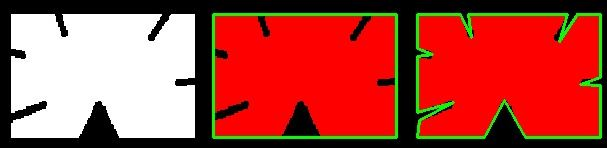5. 轮廓凸包¶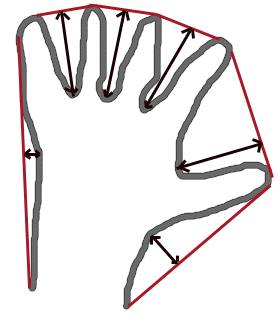hull = cv.convexHull(points[, hull[, clockwise[, returnPoints]]

hull = cv.convexHull(cnt)

6. 检查凸度¶

cv.isContourConvex()具有检查曲线是否凸出的功能。它只是返回True还是False。没什么大不了的。

k = cv.isContourConvex(cnt)

7. 边界矩形¶

7.a.直角矩形¶

(x，y)为矩形的左上角坐标，而(w，h)为矩形的宽度和高度。

x,y,w,h = cv.boundingRect(cnt)
cv.rectangle(img,(x,y),(x+w,y+h),(0,255,0),2)

7.b. 旋转矩形¶

rect = cv.minAreaRect(cnt)
box = cv.boxPoints(rect)
box = np.int0(box)
cv.drawContours(img,[box],0,(0,0,255),2)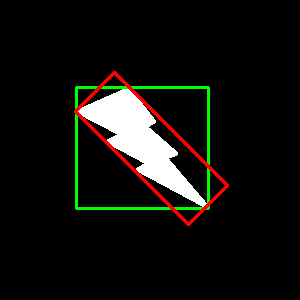8. 最小闭合圈¶

center = (int(x),int(y))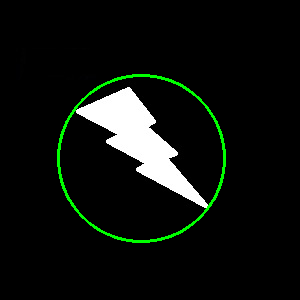9. 拟合一个椭圆¶

ellipse = cv.fitEllipse(cnt)
cv.ellipse(img,ellipse,(0,255,0),2)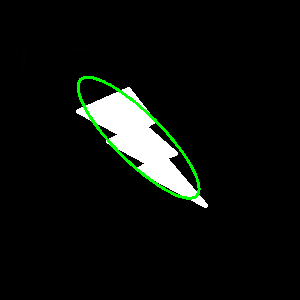10. 拟合直线¶

rows,cols = img.shape[:2]
[vx,vy,x,y] = cv.fitLine(cnt, cv.DIST_L2,0,0.01,0.01)
lefty = int((-x*vy/vx) + y)
righty = int(((cols-x)*vy/vx)+y)
cv.line(img,(cols-1,righty),(0,lefty),(0,255,0),2)